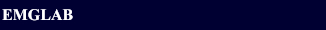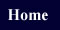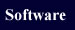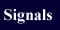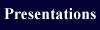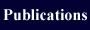EMGLAB FORUMEMGLAB FORUM >About eaf files in the dataset R008 (Multi-channel IEMG)

Subject:   About eaf files in the dataset R008 (Multi-channel IEMG)

 From:   maoqi chen Date:   29 May 2018 11:36 pm Reply via e-mail to maoqi chen.
Hi,
I had a question when looking at the eaf file of dataset R008. The multichannel signals in R008 only have up to 8 channels, but the manual decomposition results given in the eaf file always have 9 channels.
such as follows:
"
Manually decomposed by expert.
The most confident channel for each unit:
1 = c2; 2 = c4; 3 = c3; 4 = c1; 5 = c3; 6 = c2; 7 = c1; 8 = c3;
9 = c2; 10 = c2; 11 = c2; 12 = c5; 13 = c2; 14 = c0; 15 = c2; 16 = c5; 17 = c5; 18 = c1; 19 = c2; 20 = c0; 21 = c2; 22 = c5; 23 = c5; 24 = c3; 25 = c3; 26 = c8; 27 = c8; 28 = c3;
"

So what does "c0" mean?
Thanks a lot!